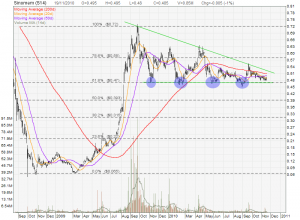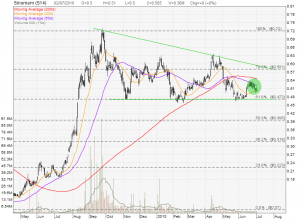## Sinomem Fundamental Analysis and Chart Pattern

• Post author:

Sinomem Fundamental Analysis

• Current Price = \$0.485
• IPO Price = \$0.44
• Current NAV = \$0.534
• Current PE = 10.5
• Rolling PE = 7.2
• Dividend Yield = 2.06%
• FY09 Net Profit Margin = 17.7%
• FY09 ROA = 6.6%
• FY09 ROE = 9.2%
• FY09 Current Ratio = 2.16
• FY09 Debt to Equity = 0.13

Sinomem Current Chart Pattern

Sinomem is currently forming a Descending Triangle with a very strong support at \$0.47 (which is also a 61.8% Fibonacci Retracement Support). Breaking below this support level will give a very bearish chart pattern for Sinomem. However, breaking out from this Descending Triangle will send Sinomem to minimum price target of \$0.72. Base on current PE ratio, this stock is not over value.Base on Fundamental & Chart Pattern Analysis, Sinomem is not for long term value investing but good for short term trading when breakout. This analysis base on my own stock selection criteria.

## Sinomem: Forming a Falling Wedge

• Post author:

Sinomem is forming a Falling Wedge and has a risk of breaking down after falling to break the 50D MA resistance (area shaded in green). Critical support at \$0.472 which is the wedge support and also 61.8% Fibonacci Retracement Level.

• Breaking this support will send Sinomem down to \$0.223 (also 23.6% Fibonacci Retracement Level).
• Upside kept at about \$0.581, which is the falling wedge’s resistance and also 78.6% FR level.